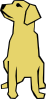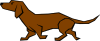Click here for the Problem Extension Worksheet version of the Problems of the Week.
Click here for a MS Word version of the Problems of the Week.
Click here for the Canadian Problem Extension Worksheet version of the Problems of the Week.
Click here for a Canadian MS Word version of the Problems of the Week.Lower Elementary:
Question: Layla takes her dog for a 20 minute walk each morning. On Monday, she takes the dog out for a walk at 7:55 AM and walks the dog for 8 minutes longer than the usual amount of time. At what time do they get back to their house?
Answer: 8:23 AM
Solution: Twenty minutes after 7:55 AM is 8:15 AM, and 8 minutes after that is 8:23 AM.Upper Elementary:
Question: Spot the dog can jump 3¾ feet into the air. Can Spot catch a frisbee that flies 44 inches over his head?
Answer: Yes
Solution: One way to solve this problem is to convert 3¾ feet to inches. Three feet is 3 × 12 = 36 inches, and a quarter of a foot is 12 ÷ 4 = 3 inches. So, 3¾ is 36 + 3 + 3 + 3 = 45 inches. Spot can jump more than 44 inches, so he can catch the frisbee.Middle School:
Question: A box of dog biscuits is 10 centimeters long, 5 centimeters wide, and 18 centimeters tall. Each dog biscuit takes up 2 cubic centimeters of space in the box. How many dog biscuits can fit in the box?
Answer: 450 biscuits
Solution: The box is 10 × 5 × 18 = 900 cubic centimeters in volume. That means that it can hold 900 ÷ 2 = 450 biscuits.Algebra and Up:
Question: Steven uses the equation below to find the average change in weight of his dogs after a month of feeding them Such Healthy Very Dog Wow brand dog food.

(2 – 3 + 1 + 5 + 4 + 6) ÷ 8 = x

What is the data set demonstrated by the equation?
Answer: [–3, 0, 0, 1, 2, 4, 5, 6]
Solution: When we find an average of a set, we add up all the values in the set and divide the sum by the number of values. The data set therefore includes all the numbers in the numerator above. But hang on! We’re dividing by 8, but there are 6 values added together. That means that there must have been two additional dogs whose weight loss was 0 because Steven could have skipped them in his equation and the average wouldn’t change.